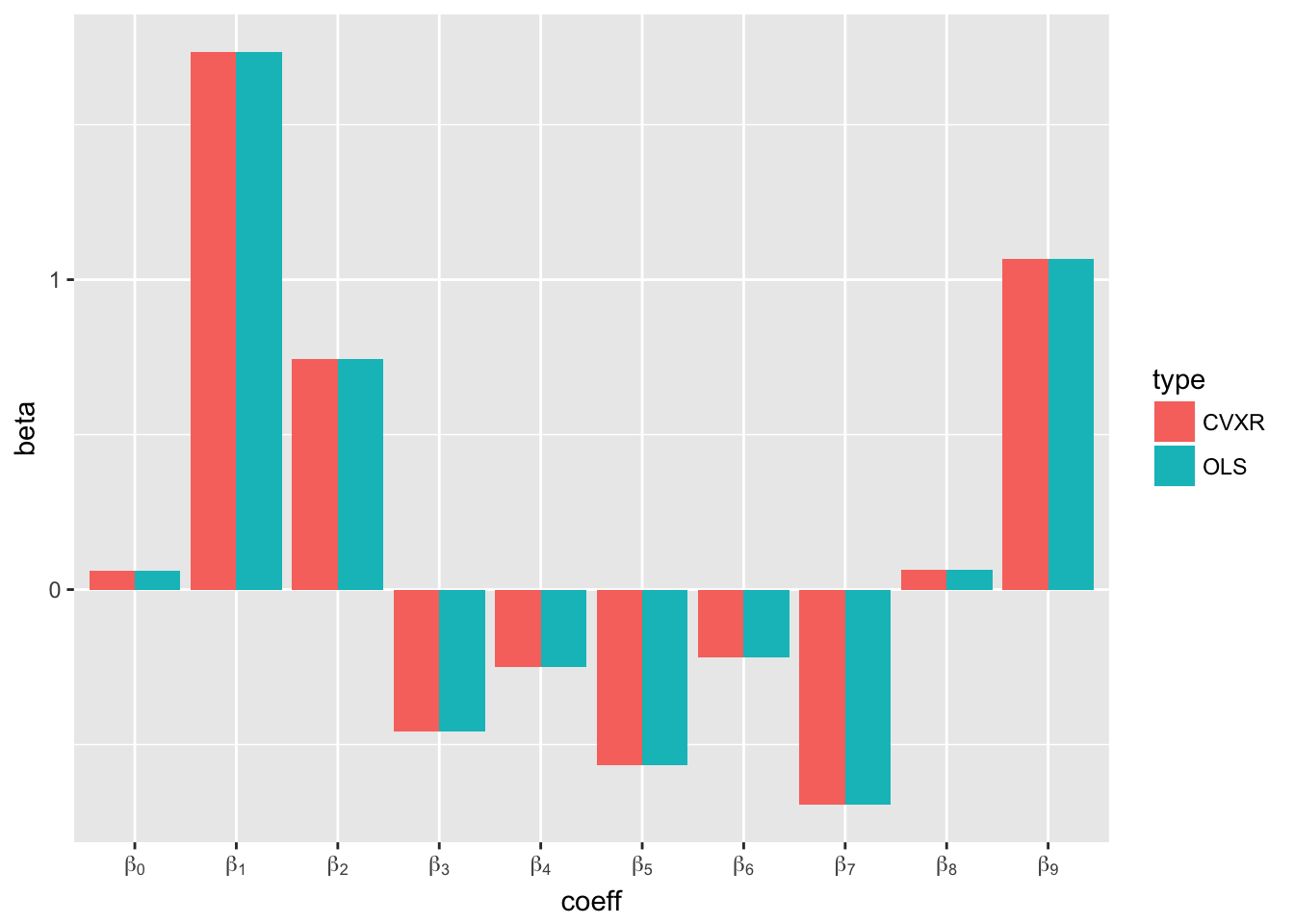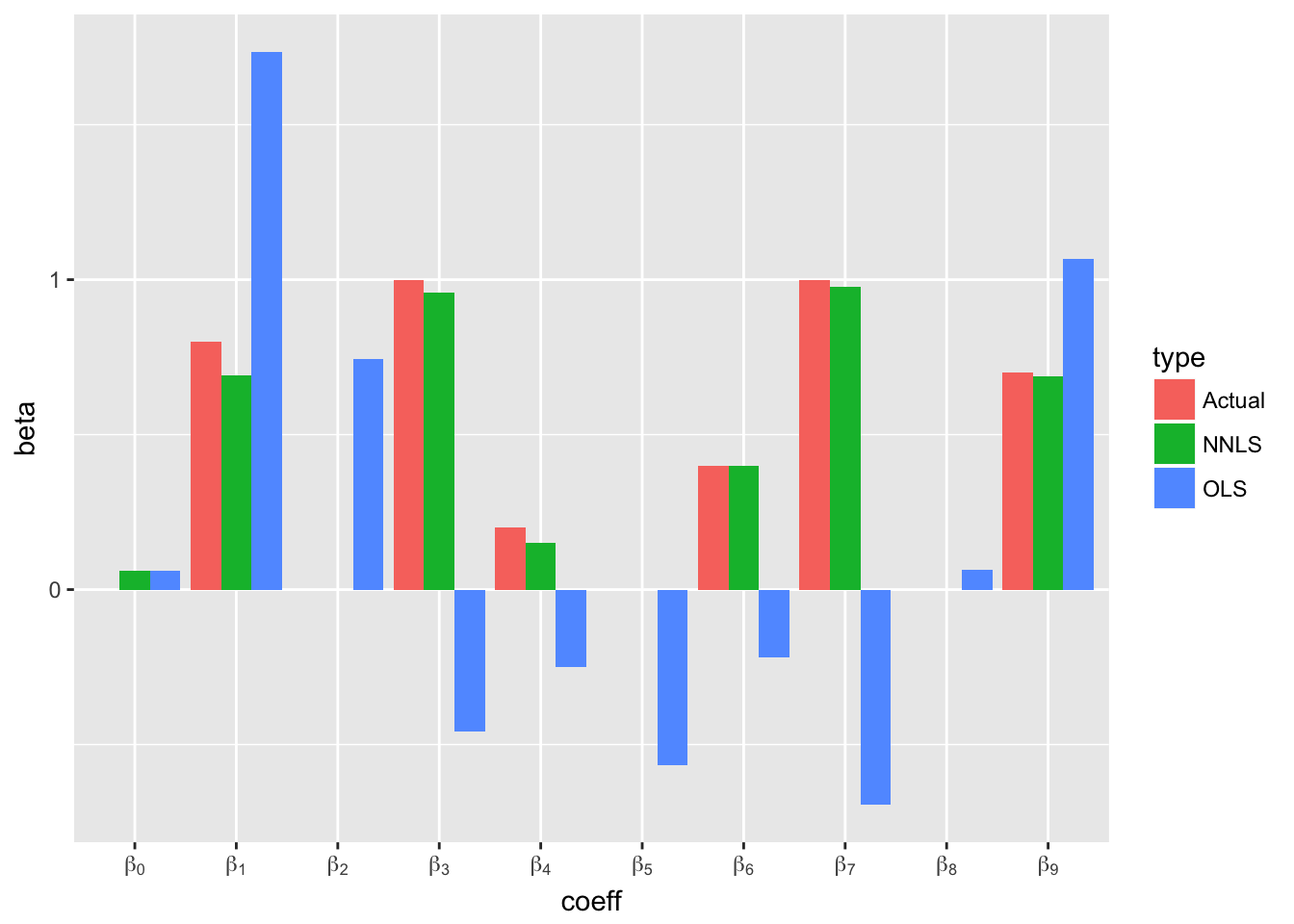# CVXR: An R Package for Disciplined Convex Optimization

by Anqi Fu, Balasubramanian Narasimhan, Stephen Boyd

At long last, we are pleased to announce the release of CVXR!

First introduced at useR! 2016, CVXR is an R package that provides an object-oriented language for convex optimization, similar to CVX, CVXPY, YALMIP, and Convex.jl. It allows the user to formulate convex optimization problems in a natural mathematical syntax, then automatically verifies the problem’s convexity with disciplined convex programming (DCP) and converts it into the appropriate form for a specific solver. This makes CVXR ideal for rapidly prototyping new statistical models. More information is available at the official site.

This is the first of a series of blog posts about CVXR. In this post, we will introduce the semantics of our package and dive into a simple example, which gives users an idea of CVXR’s power.

## Convex Optimization

A convex optimization problem has the form $\begin{array}{ll} \underset{v}{\mbox{minimize}} & f_0(v)\\ \mbox{subject to} & f_i(v) \leq 0, \quad i=1,\ldots,M\\ & g_i(v) = 0, \quad i=1,\ldots,P \end{array}$ where $$v$$ is the variable, $$f_0$$ and $$f_1,...,f_m$$ are convex, and $$g_1,...,g_p$$ are affine. In CVXR, variables, expressions, objectives, and constraints are all represented by S4 objects. Users define a problem by combining constants and variables with a library of basic functions (atoms) provided by the package. When solve() is called, CVXR converts the S4 object into a standard form, sends it to the user-specified solver, and retrieves the results. Let’s see an example of this in action.

## Ordinary Least Squares (OLS)

We begin by generating data for an ordinary least squares problem.

set.seed(1)
s <- 1
n <- 10
m <- 300
mu <- rep(0, 9)
Sigma <- cbind(c(1.6484, -0.2096, -0.0771, -0.4088, 0.0678, -0.6337, 0.9720, -1.2158, -1.3219),
c(-0.2096, 1.9274, 0.7059, 1.3051, 0.4479, 0.7384, -0.6342, 1.4291, -0.4723),
c(-0.0771, 0.7059, 2.5503, 0.9047, 0.9280, 0.0566, -2.5292, 0.4776, -0.4552),
c(-0.4088, 1.3051, 0.9047, 2.7638, 0.7607, 1.2465, -1.8116, 2.0076, -0.3377),
c(0.0678, 0.4479, 0.9280, 0.7607, 3.8453, -0.2098, -2.0078, -0.1715, -0.3952),
c(-0.6337, 0.7384, 0.0566, 1.2465, -0.2098, 2.0432, -1.0666,  1.7536, -0.1845),
c(0.9720, -0.6342, -2.5292, -1.8116, -2.0078, -1.0666, 4.0882,  -1.3587, 0.7287),
c(-1.2158, 1.4291, 0.4776, 2.0076, -0.1715, 1.7536, -1.3587, 2.8789, 0.4094),
c(-1.3219, -0.4723, -0.4552, -0.3377, -0.3952, -0.1845, 0.7287, 0.4094, 4.8406))
X <- mvrnorm(m, mu, Sigma)
X <- cbind(rep(1, m), X)
trueBeta <- c(0, 0.8, 0, 1, 0.2, 0, 0.4, 1, 0, 0.7)
y <- X %*% trueBeta + rnorm(m, 0, s)

Here, n is the number of predictors, y is the response, and X is the matrix of predictors. In CVXR, we first instantiate the optimization variable.

beta <- Variable(n)

beta is a Variable S4 object, which does not contain a value yet.

In the next line, we define the objective function.

objective <- Minimize(sum((y - X %*% beta)^2))

The expression sum((y - X %*% beta)^2) is another S4 object, created by combining y, X, and beta using the basic addition, subtraction, multiplication, and power atoms. Thus, the call to Minimize() does not return its minimum value (after all, beta doesn’t have a value yet, so we cannot evaluate the expression), but simply defines the goal of our optimization problem.

Finally, we construct the Problem and call solve(), which invokes the default ECOS solver.

prob <- Problem(objective)
CVXR_result <- solve(prob)

This returns a list containing, among other things, the solver status, objective value, and function getValue() that takes as input a Variable and retrieves its optimal value from the solution.

CVXR_result$status # solution status by solver ##  "optimal" CVXR_result$value            # optimal objective value
##  340.3187
cvxrBeta <- CVXR_result$getValue(beta) # optimal value of beta Below, we plot the CVXR coefficients beside the coefficients found by lm(). p <- length(trueBeta) lm_result <- lm(y ~ 0 + X) lmBeta <- coef(lm_result) df <- data.frame(coeff = rep(paste0("beta[", seq_along(lmBeta) - 1L, "]"), 2), beta = c(lmBeta, cvxrBeta), type = c(rep("OLS", p), rep("CVXR", p))) ggplot(data = df, mapping = aes(x = coeff, y = beta)) + geom_bar(mapping = aes(fill = type), stat = "identity", position = "dodge") + scale_x_discrete(labels = parse(text = levels(df$coeff)))As expected, they are identical. Obviously, if all you want to fit is OLS, you should use lm(). The chief advantage of CVXR is its ability to quickly modify and adapt a problem, as we illustrate in the next section.

### Non-Negative Least Squares (NNLS)

In many situations, we can greatly improve our model by constraining the solution to reflect our prior knowledge. For example, we may know that beta must be non-negative. We can easily incorporate this knowledge into our problem by passing an additional argument to the Problem() constructor, which specifies a list of constraints.

prob2 <- Problem(objective, list(beta >= 0))

Again, counter to what you might expect, the expression beta >= 0 does not return TRUE or FALSE the way 1.3 >= 0 would. Instead, the == and >= operators have been overloaded to return Constraint S4 objects, which will be used by the solver to enforce the problem’s constraints. We then re-solve the new problem with the non-negativity constraint.

CVXR_result2 <- solve(prob2)
cvxrBetaNNLS <- CVXR_result2$getValue(beta) As you can see in the figure below, adding that one constraint produced a massive improvement in the accuracy of the estimates. Not only are the NNLS estimates much closer to the true coefficients than the OLS estimates, but they have even managed to recover the correct sparsity structure in this case. df <- data.frame(coeff = rep(paste0("beta[", seq_along(trueBeta) - 1L, "]"), 3), beta = c(trueBeta, lmBeta, cvxrBetaNNLS), type = c(rep("Actual", p), rep("OLS", p), rep("NNLS", p))) ggplot(data = df, mapping = aes(x = coeff, y = beta)) + geom_bar(mapping = aes(fill = type), stat = "identity", position = "dodge") + scale_x_discrete(labels = parse(text = levels(df$coeff)))Like with OLS, there are already R packages available that implement NNLS. But that is actually an excellent demonstration of the power of CVXR: A single line of code here - namely, prob2 <- Problem(objective, list(beta >= 0)) - is doing the work of an entire package.

We hope this gives you an idea of the power of CVXR. In our next post, we will explore a non-parametric estimation problem that introduces more atoms and constraints.

Share Comments · · · ·